Name: ___________________Date:___________________

 Email us to get an instant 20% discount on highly effective K-12 Math & English kwizNET Programs!

### MEAP Preparation - Grade 4 Mathematics1.41 Types of Fractions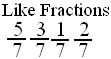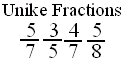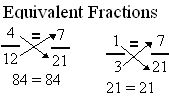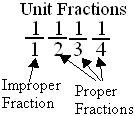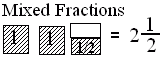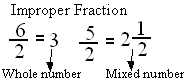Directions: Answer the following questions. Also write at least 5 examples of each type of fractions.Name: ___________________Date:___________________

### MEAP Preparation - Grade 4 Mathematics1.41 Types of Fractions

 Q 1: When a products obtained by cross multiplication give the same number, then the fractions are ____(or equal).equivalentpropermixed Q 2: Fractions with different denominators are calledunlike fractionslike fractions Q 3: A number with a whole number and a proper fraction is aproper numbermixed number Q 4: A mixed number denotes the sum of a whole number-part and proper fraction part.FalseTrue Q 5: An improper fraction is sometimes a mixed number or sometimes a whole number.FalseTrue Q 6: Fractions with same denominators are calledunlike fractionslike fractions Q 7: If the numerator is more than the denominator in a fraction, the fraction is called a _________.mixed fractionimproper fractionproper fraction Q 8: If the numerator is less than the denominator in a fraction, the fraction is called a _______.mixed fractionimproper fractionproper fraction Question 9: This question is available to subscribers only! Question 10: This question is available to subscribers only!